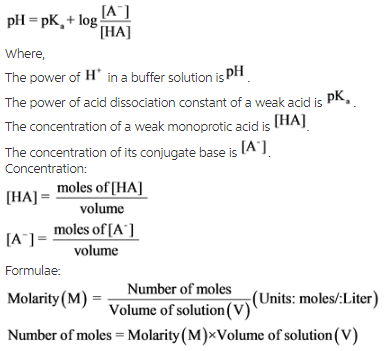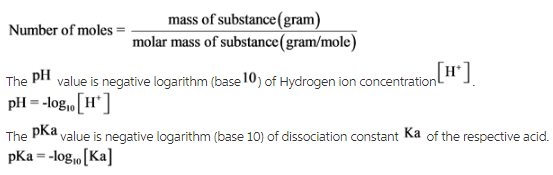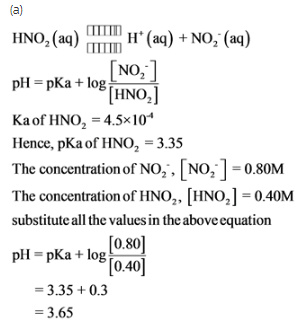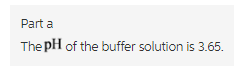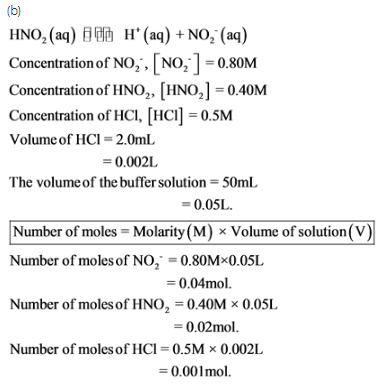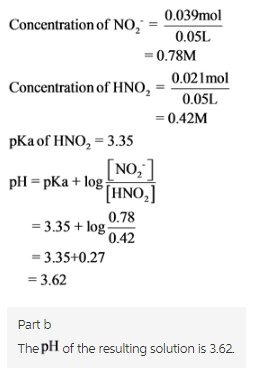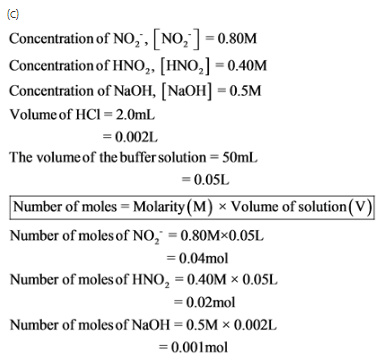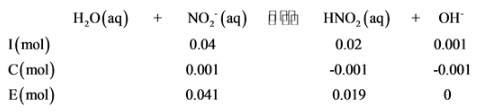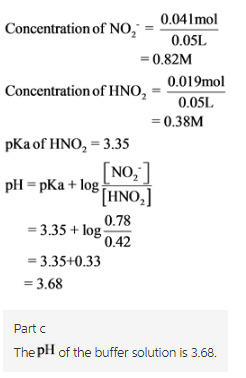# Nitric acid, HNO2 is a weak acid with Ka = 4.5 x 10-4. It dissociates according to: HNO2 (aq)

Nitric acid, HNO2 is a weak acid with Ka = 4.5 x 10-4. It dissociates according to:
HNO2 (aq) <–> H+ (aq) + NO2- (aq)

a.) What is the pH of a buffer solution which is 0.80 M in NO2- and 0.40 M in HNO2?
b.) What will be the pH of the resulting solution when 2.0 mL of 0.5 M HCl are added to 50 mL of this (from part a) buffer solution?
c.) What will be the pH of the resulting solution when 2.0 mL of 0.5 M NaOH are added to 50 mL of this (from part a) buffer solution?

An answer accompanied by a complete, step by step solution would be greatly appreciated.

Concepts and reason
Buffer:

A buffer is a solution that contains a weak acid/base mixed with its conjugate base/acid (as in the form of salts). It can resist PH change when a small amount of a strong acid/base is added to it.
Solutions are classified into acids or bases based on the pH value.
pH is a measure of hydrogen ion present in a solution. It is the negative logarithm of the H+ concentration to the base 10. It gives the acidic strength of the solution.

Fundamentals
Henderson-Hasselbalch equation is a mathematical expression which can be used to calculate the PH of buffer solutions.